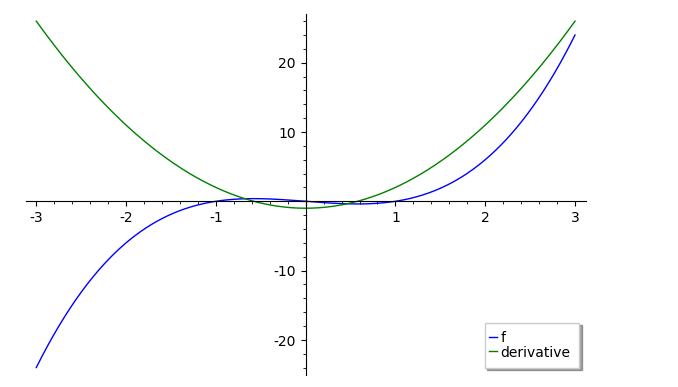# Revision history [back]

### plotted real intersection but solve only shows imaginary

I plotted x^3-x and its derivative 3x^2 - 1 to get two intersections in the real plane. However, when I solved the two with solve(x^3-x == 3x^2 - 1,x), all I get are imaginary values. Shouldn't Solve show me the two intersections or am I disastrously confused?

[x == -1/2*(1/9*I*sqrt(37)*sqrt(3) + 1)^(1/3)*(I*sqrt(3) + 1) - 2/3*(-I*sqrt(3) + 1)/(1/9*I*sqrt(37)*sqrt(3) + 1)^(1/3) + 1, x == -1/2*(1/9*I*sqrt(37)*sqrt(3) + 1)^(1/3)*(-I*sqrt(3) + 1) - 2/3*(I*sqrt(3) + 1)/(1/9*I*sqrt(37)*sqrt(3) + 1)^(1/3) + 1, x == (1/9*I*sqrt(37)*sqrt(3) + 1)^(1/3) + 4/3/(1/9*I*sqrt(37)*sqrt(3) + 1)^(1/3) + 1]


### plotted real intersection but solve only shows imaginary

I plotted x^3-x and its derivative 3x^2 - 1 to get two intersections in the real plane. However, when I solved the two with solve(x^3-x == 3x^2 - 1,x), all I get are imaginary values. Shouldn't Solve show me the two intersections or am I disastrously confused?

plot(x^3-x,-3,3,color='blue',legend_label="f") + plot(
3*x^2 - 1,-3,3,color='green',legend_label="derivative")

[x == -1/2*(1/9*I*sqrt(37)*sqrt(3) + 1)^(1/3)*(I*sqrt(3) + 1) - 2/3*(-I*sqrt(3) + 1)/(1/9*I*sqrt(37)*sqrt(3) + 1)^(1/3) + 1, x == -1/2*(1/9*I*sqrt(37)*sqrt(3) + 1)^(1/3)*(-I*sqrt(3) + 1) - 2/3*(I*sqrt(3) + 1)/(1/9*I*sqrt(37)*sqrt(3) + 1)^(1/3) + 1, x == (1/9*I*sqrt(37)*sqrt(3) + 1)^(1/3) + 4/3/(1/9*I*sqrt(37)*sqrt(3) + 1)^(1/3) + 1]


### plotted real intersection but solve only shows imaginary

I plotted x^3-x and its derivative 3x^2 - 1 to get two intersections in the real plane. However, when I solved the two with solve(x^3-x == 3x^2 - 1,x), all I get are imaginary values. Shouldn't Solve show me the two intersections or am I disastrously confused?

plot([x^3-x,3*x^2 - 1],-3,3,color=['blue','green'],legend_label=["f","derivative"])

plot(x^3-x,-3,3,color='blue',legend_label="f") + plot(
3*x^2 - 1,-3,3,color='green',legend_label="derivative")

[x == -1/2*(1/9*I*sqrt(37)*sqrt(3) + 1)^(1/3)*(I*sqrt(3) + 1) - 2/3*(-I*sqrt(3) + 1)/(1/9*I*sqrt(37)*sqrt(3) + 1)^(1/3) + 1, x == -1/2*(1/9*I*sqrt(37)*sqrt(3) + 1)^(1/3)*(-I*sqrt(3) + 1) - 2/3*(I*sqrt(3) + 1)/(1/9*I*sqrt(37)*sqrt(3) + 1)^(1/3) + 1, x == (1/9*I*sqrt(37)*sqrt(3) + 1)^(1/3) + 4/3/(1/9*I*sqrt(37)*sqrt(3) + 1)^(1/3) + 1]


### plotted real intersection but solve only shows imaginary

I plotted x^3-x and its derivative 3x^2 - 1 to get two intersections in the real plane. However, when I solved the two with solve(x^3-x == 3x^2 - 1,x), all I get are imaginary values. Shouldn't Solve show me the two intersections or am I disastrously confused?

plot([x^3-x,3*x^2 - 1],-3,3,color=['blue','green'],legend_label=["f","derivative"])

solve(x^3-x == 3x^2 - 1,x)

[x == -1/2*(1/9*I*sqrt(37)*sqrt(3) + 1)^(1/3)*(I*sqrt(3) + 1) - 2/3*(-I*sqrt(3) + 1)/(1/9*I*sqrt(37)*sqrt(3) + 1)^(1/3) + 1, x == -1/2*(1/9*I*sqrt(37)*sqrt(3) + 1)^(1/3)*(-I*sqrt(3) + 1) - 2/3*(I*sqrt(3) + 1)/(1/9*I*sqrt(37)*sqrt(3) + 1)^(1/3) + 1, x == (1/9*I*sqrt(37)*sqrt(3) + 1)^(1/3) + 4/3/(1/9*I*sqrt(37)*sqrt(3) + 1)^(1/3) + 1]
1][C:\fakepath\intersection.PNG](/upfiles/16024461625093012.png)5 None

### plotted real intersection but solve only shows imaginary

I plotted x^3-x x^3 - x and its derivative 3x^2 3*x^2 - 1 1 to get two intersections in the real plane. plane.

plot([x^3-x,3*x^2 - 1],-3,3,color=['blue','green'],legend_label=["f","derivative"])However, when I solved the two with solve(x^3-x == 3x^2 3*x^2 - 1,x), 1, x), all I get are imaginary values. Shouldn't Solve show me the two intersections or am I disastrously confused?

plot([x^3-x,3*x^2 - 1],-3,3,color=['blue','green'],legend_label=["f","derivative"])

solve(x^3-x == 3x^2 - 1,x)values.

solve(x^3-x == 3x^2 - 1,x)

[x == -1/2*(1/9*I*sqrt(37)*sqrt(3) + 1)^(1/3)*(I*sqrt(3) + 1) - 2/3*(-I*sqrt(3) + 1)/(1/9*I*sqrt(37)*sqrt(3) + 1)^(1/3) + 1, x == -1/2*(1/9*I*sqrt(37)*sqrt(3) + 1)^(1/3)*(-I*sqrt(3) + 1) - 2/3*(I*sqrt(3) + 1)/(1/9*I*sqrt(37)*sqrt(3) + 1)^(1/3) + 1, x == (1/9*I*sqrt(37)*sqrt(3) + 1)^(1/3) + 4/3/(1/9*I*sqrt(37)*sqrt(3) + 1)^(1/3) + 1][C:\fakepath\intersection.PNG](/upfiles/16024461625093012.png)
1]


Shouldn't solve show me the two intersections or am I disastrously confused?